This article describes an analogue for functions of multiple variables of the following term/fact/notion for functions of one variable: derivative

Definition at a point

Generic definition

Suppose$f$ is a function of many variables. We can view$f$ as a function of a vector variable. The gradient vector at a particular point in the domain is a vector whose direction captures the direction (in the domain) along which changes to$f$ are concentrated, and whose magnitude is the directional derivative in that direction.

If the gradient vector of$f$ exists at a point, then we say that$f$ is differentiable at that point.

Formal epsilon-delta definition

Suppose$f$ is a function of a vector variable$\overline{x}$. Suppose$\overline{c}$ is a point in the interior of the domain of$f$, i.e.,$f$ is defined in an open ball centered at$\overline{c}$. The gradient vector of$f$ at$\overline{c}$, denoted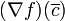$(\nabla f)(\overline{c})$, is a vector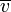$\overline{v}$ satisfying the following:

• For every$\varepsilon > 0$
• there exists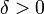$\delta > 0$ such that
• for every$\overline{x}$ satisfying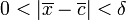$0 < |\overline{x} - \overline{c}| < \delta$ (in other words,$\overline{x}$ is in an open ball of radius$\delta$ centered at$\overline{c}$, but not qual to$\overline{c}$)
• we have$|f(\overline{x}) - f(\overline{c}) - \overline{v} \cdot (\overline{x} - \overline{c})| < \varepsilon|\overline{x} - \overline{c}|$

Note on why the epsilon-delta definition is necessary

Intuitively, we want to define the gradient vector analogously to the derivative of a function of one variable, i.e., as the limit of the difference quotient: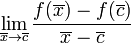$\lim_{\overline{x} \to \overline{c}} \frac{f(\overline{x}) - f(\overline{c})}{\overline{x} - \overline{c}}$

Unfortunately, the above notation does not make direct sense because it is not permissible to divide a scalar by a vector. To rectify this, we revisit what the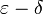$\varepsilon-\delta$ definition of the derivative says. It turns out that that$\varepsilon-\delta$ definition can more readily be generalized to functions of vector variables. The key insight is to use the dot product of vectors.

Definition as a function

Generic definition

Suppose$f$ is a function of many variables. We can view$f$ as a function of a vector variable. The gradient vector of$f$ is a vector-valued function (with vector outputs in the same dimension as vector inputs) defined as follows: it sends every point to the gradient vector of the function at the point. Note that the domain of the function is precisely the subset of the domain of$f$ where the gradient vector is defined.

If the gradient vector of$f$ exists at all points of the domain of$f$, we say that$f$ is differentiable everywhere on its domain.

Relation with directional derivatives and partial derivatives

Relation with directional derivatives

For further information, refer: Relation between gradient vector and directional derivatives

Version type Statement
at a point, in vector notation (multiple variables) Suppose$f$ is a function of a vector variable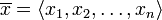$\overline{x} = \langle x_1,x_2,\dots,x_n \rangle$. Suppose$\overline{u}$ is a unit vector and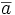$\overline{a}$ is a point in the domain of$f$. Suppose that the gradient vector of$f$ at$\overline{a}$ exists. We denote this gradient vector by$\nabla f(\overline{a})$. Then, we have the following relationship: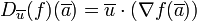$D_{\overline{u}}(f)(\overline{a}) = \overline{u} \cdot (\nabla f(\overline{a}))$
The right side here is the dot product of vectors.
generic point, in vector notation (multiple variables) Suppose$f$ is a function of a vector variable$\overline{x} = \langle x_1,x_2,\dots,x_n \rangle$. Suppose$\overline{u}$ is a unit vector. We then have: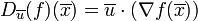$D_{\overline{u}}(f)(\overline{x}) = \overline{u} \cdot (\nabla f(\overline{x}))$
The right side here is a dot product of vectors. The equality holds whenever the right side makes sense.
generic point, point-free notation (multiple variables) Suppose$f$ is a function of a vector variable$\overline{x} = \langle x_1,x_2,\dots,x_n \rangle$. Suppose$\overline{u}$ is a unit vector. We then have: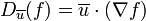$D_{\overline{u}}(f) = \overline{u} \cdot (\nabla f)$
The right side here is a dot product of vector-valued functions (the constant function$\overline{u}$ and the gradient vector of$f$). The equality holds whenever the right side makes sense.

Relation with partial derivatives

For further information, refer: Relation between gradient vector and partial derivatives

Version type Statement
at a point, in multivariable notation Suppose$f$ is a real-valued function of$n$ variables$x_1,x_2,\dots,x_n$. Suppose$(a_1,a_2,\dots,a_n)$ is a point in the domain of$f$ such that the gradient vector of$f$ at$(a_1,a_2,\dots,a_n)$, denoted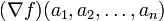$(\nabla f)(a_1,a_2,\dots,a_n)$, exists. Then, the partial derivatives of$f$ with respect to all variables exist, and the coordinates of the gradient vector are the partial derivatives. In other words:$(\nabla f)(a_1,a_2,\dots,a_n) = \langle f_{x_1}(a_1,a_2,\dots,a_n), f_{x_2}(a_1,a_2,\dots,a_n), \dots f_{x_n}(a_1,a_2,\dots,a_n)\rangle$
generic point, in multivariable notation Suppose$f$ is a real-valued function of$n$ variables$x_1,x_2,\dots,x_n$. Then, we have$(\nabla f)(x_1,x_2,\dots,x_n) = \langle f_{x_1}(x_1,x_2,\dots,x_n), f_{x_2}(x_1,x_2,\dots,x_n), \dots f_{x_n}(x_1,x_2,\dots,x_n)\rangle$.
Equality holds wherever the left side makes sense.
generic point, point-free notation Suppose$f$ is a function of$n$ variables$x_1,x_2,\dots,x_n$. Then, we have$\nabla f = \langle f_{x_1}, f_{x_2}, \dots f_{x_n} \rangle$. Equality holds wherever the left side makes sense.

Note on continuous partials

For further information, refer: Continuous partials implies differentiable

This says that if all the partial derivatives of a function are continuous at and around a point in the domain, then the function is in fact differentiable, hence the gradient vector is described in terms of the partial derivatives as described above.

In particular, if all the partials exist and are continuous everywhere, the gradient vector exists everywhere and is given as described above.

Note that this is significant because, a priori (i.e., without checking continuity), knowledge of the partials tells us what the gradient vector should be if it exists, but it doesn't tell us whether the gradient vector does exist. Continuity helps bridge that knowledge gap.

Graphical interpretation

For a function of two variables

Suppose$f$ is a function of two variables$x,y$ and suppose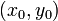$(x_0,y_0)$ is a point in the domain. We say that$f$ is differentiable at a point$(x_0,y_0)$ if the gradient vector exists at the point. This is equivalent to the graph of the function having a well defined tangent plane at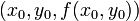$(x_0,y_0,f(x_0,y_0))$. Further, the equation of this tangent plane is given by: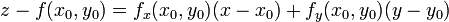$z - f(x_0,y_0) = f_x(x_0,y_0)(x - x_0) + f_y(x_0,y_0)(y - y_0)$

Another way of putting this is:$z - f(x_0,y_0) = (\nabla f)(x_0,y_0) \cdot (\langle x,y \rangle - \langle x_0,y_0\rangle)$

Note that it is possible that the partial derivatives both exist but the function is not differentiable. In this case, the surface does not have a well defined tangent plane at the point. Even though we can define a plane by the equation above, this is not the tangent plane, because the tangent plane does not exist.

For a function of multiple variables

Suppose$f$ is a function of multiple variables$x_1,x_2,\dots,x_n$ and suppose$(a_1,a_2,\dots,a_n)$ is a point in the domain of$f$. We say that$f$ is differentiable at$(a_1,a_2,\dots,a_n)$ if the gradient vector$(\nabla f)(a_1,a_2,\dots,a_n)$ exists. This is equivalent to the graph of the function having a well defined tangent hyperplane at the point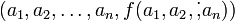$(a_1,a_2,\dots,a_n,f(a_1,a_2,\dot,a_n))$. The equation of the tangent hyperplane is given by:$x_{n+1} - f(a_1,a_2,\dots,a_n) = (\nabla f)(a_1,a_2,\dots,a_n) \cdot (\langle x_1,x_2,\dots,x_n\rangle - \langle a_1,a_2,\dots,a_n \rangle)$